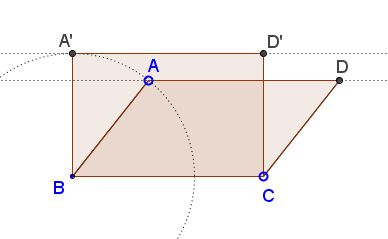# Isoperimetric Theorem for Parallelograms

### Rectangle among Parallelograms

For any parallelogram (which is not a rectangle) there is a rectangle of the same perimeter but larger area.Indeed, according to Euclid I.35, parallelograms with the same base and in the same parallels have equal areas. Restricting to rectangles, it is clear that, among rectangles with the same base, the area grows with the distance between the parallel lines. Since in a circle, the point farthest from a diameter, lies on the perpendicular radius, the area of a rectangle among all parallelograms with the same sides is the largest.

### Acknowledgment

The above has been communicated to me in private correspondence by Sidney Kung on 1 March, 2014.

• Isoperimetric Theorem and Inequality
• An Isoperimetric theorem
• Isoperimetric theorem and its variants
• Isoperimetric Property of Equilateral Triangles
• Isoperimetric Property of Equilateral Triangles II
• Maximum Area Property of Equilateral Triangles
•